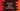# How to return objects from JavaScript functions## How to return objects from JavaScript functions:

JavaScript functions can return values or they can return nothing or undefined. But, what about objects? Can we return objects from functions in JavaScript? In this post, we will learn how to return objects from JavaScript functions.I will also show you how functions return values.

### Returning values from a JavaScript function:

Let’s take a look at the example below:

``````function giveMeAString() {
return 'Hello';
}

function giveMeANumber() {
return 2;
}

function giveMeABoolean() {
return true;
}

function giveMeNothing() {
return;
}

function donotReturn() {
}

console.log(giveMeAString());
console.log(giveMeANumber());
console.log(giveMeABoolean());
console.log(giveMeNothing());
console.log(donotReturn());``````

Here,

• giveMeAString function returns a string.
• giveMeANumber function returns a number.
• giveMeABoolean function returns a boolean.
• giveMeNothing function returns nothing.
• donotReturn function don’t have a return statement.

We are printing the responses of all of these functions. If you run this program, it will print the below output:

``````Hello
2
true
undefined
undefined``````

So, you can see here that it prints undefined or the function returns undefined if it is not returning any value or if it don’t have the return statement.

### Returning objects from functions in JavaScript:

Returning objects from functions is similar to returning anything else from a function. Instead of returning a different value, you are returning an object.

Let me show you an example:

``````function getError(m, c) {
return {msg: m, code: c};
}

let e = getError('Bad request !!', 400);

console.log(`Error: \${e.msg}, code: \${e.code}`);``````

In this example, we have created a function getError that returns one object. It takes two parameters and returns one object. The log statement is printing the content of the object.

If you run the above program, it will print the below output:

``Error: Bad request !!, code: 400``

### Returning a function:

Let’s take another example on how to return a function from a function:

``````function getError(m, c) {
return {msg: m, code: c};
}

function getResult(type, m, c){
switch(type){
case 'error':
return getError(m,c);
default:
return;
}
}

let e = getResult('error', 'Bad request !!', 400);

console.log(`Error: \${e.msg}, code: \${e.code}`);``````

In this example, we are calling getResult and it returns getError. getError is a function and that returns an object. So, basically, getResult is returning an object if the type is error.

If you run this program, it will print the same output.